Search IntMath
Close

450+ Math Lessons written by Math Professors and Teachers

5 Million+ Students Helped Each Year

1200+ Articles Written by Math Educators and Enthusiasts

Simplifying and Teaching Math for Over 23 Years

# Explore the slope of the sin curve

By Murray Bourne, 25 Oct 2010

UPDATE: I've created a new interactive trigonometry derivatives applet to replace the out-of-date one that was on this page.

The background below is still worth reading!

## Background

This was an important problem for mathematicians for centuries. If something is moving so that its velocity and acceleration are forever changing, how do we measure the rate of change?

It is easy to find rate of change (or slope, or gradient) for an object moving at constant speed, or constant acceleration, but what do we do if when the speed or acceleration is not constant, as is the case for objects moving in circular or regular paths (which we can describe using the trigonometric functions)?

This article, and the 2 that follow, shed some light on this interesting problem.

First, some reminders so you can better follow what is going on.

## Tangent to a curve

A tangent to a curve means the line that touches the curve at one point only.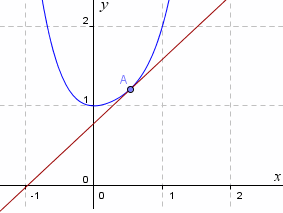The tangent of the curve at the point A (screen shot)

The slope of a curve means the slope of the tangent at a particular point.

## Curve Reminders

Here is the curve y = sin x. The values of x are in radians and one complete cycle goes from 0 to (or around 6.28).

#### Graph of sin x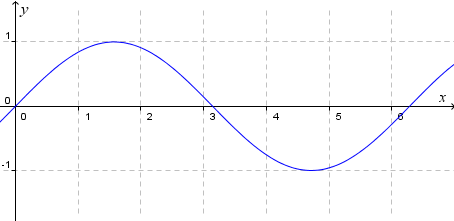y = sin x

Our aim is to explore the slope of the curve y = sin x. Clearly. the slope of the curve changes as we move left to right.

#### Graph of cos x

Next we have y = cos x. It has the same shape as the sine curve, but has been displaced (shifted) to the left by π/2 (or 90°).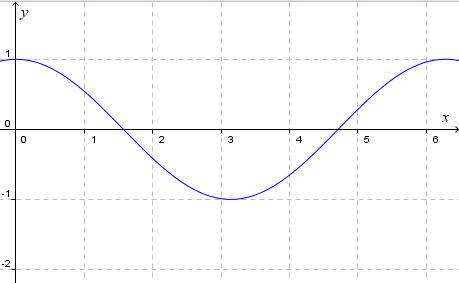y = cos x

## Slope of Sine x

First, have a look at the interactive graph below and observe that the slope of the (red) tangent line at the point A is the same as the y-value of the point B.

Then slowly drag the point A right or left and observe the curve traced out by point B. (The point B has the same x-value as point A, and its y-value is the same as the slope of the curve at point A).

Update: This applet has been replaced with a new one here:

Hopefully you can see that B traces out the curve y = cos x. In other words, the slope of the graph y = sin x at any point (x,y) has value cos x. Using calculus notation, we would write this as: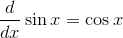In the following 2 related articles, we examine the slope of y = cos x and learn about the slope of the curve y = tan x.

See more on the differentiation of sin, cos and tan curves. (This is in the calculus section of IntMath.)

### 16 Comments on “Explore the slope of the sin curve”

1. Sue VanHattum says:

>A tangent to a curve means the line that touches the curve at one point only.

One of the things I discuss with students in calculus is how hard it is to come up with a good definition of tangent. Tangents lines can touch the curve more than once. (Most tangents to y=x^3 will cross somewhere else. And the tangent to y=sin x and x=pi/2 touches an infinite number of times.)

Students will also say the tangent doesn't cross the curve, but that caveat is violated whenever you want a tangent at an inflection point.

A good discussion can be had by asking how this wrong definition can be fixed. Zooming in, we find that the tangent will only touch the curve once within a "small enough" interval, which brings us back once again to limits. That's still not enough, though...

2. Murray says:

Hi Sue and thanks for the comment. Yes, I normally say a tangent is defined "within a local region" but decided not to go there for the sake of this short article.

3. gagangc says:

sir,
this interactive graph helps,
but how will u find the slope of a function that is double valued at a point? for example how to find slope of ellipse or circle centered at origin?

4. Murray says:

@gagan: Actually, strictly there is no slope of an ellipse or circle, since they are not functions (there is more than one y-value for each x-value).

But we can take half of each shape (say the top half) and go ahead and find the slope using derivatives.

5. josh says:

how do you work out the rate of change on a curve

6. Murray says:

@Josh: See this Introduction to Differentiation and the sections following that. Hope it helps!

7. Ed Watts says:

I cannot use the Geogbra link on this page can you please assist me as I do not wish to use Firefox. Any help appreciated

8. Murray says:

Hi Ed. Unfortunately, like many other things, Internet Explorer won't show the GeoGebra-base JSXgraph on that page. You can use any quality browser, like Safari or Chrome, if Firefox is not an option. Good luck with it!

9. darshan kumar says:

wht is physical meaning of d^2x/d^2y

10. Murray says:

@Darshan: The second derivative tells us the rate that the first derivative is changing.

Take displacement (x), velocity (dx/dt) and acceleration (d^2x/dt^2).

Velocity tells us the rate displacement is changing at a particular time. (High velocity means the displacement is changing very quickly per time unit).

Acceleration tells us the rate the velocity is changing. (High acceleration means the velocity is changing quickly per time unit.)

Hope that helps.

11. priyoranjan says:

dear sir,

could you help me find out the value of
sin1+sin2+sin3+sin4+......+sin90
i worked it out on a calculator which came out to be 57.794322 but i wanted a way to develop a formula which would help me out.

12. Murray says:

@priyoranjan: I presume your angles are in degrees.

How accurate do you need your answer? You can always use calculus to get a good approximation. (See this solution using Wolfram|Alpha).

I don't know of any formula for summing sines like this.

13. sourav sharma says:

murray you can use ap(arithmetic progression) in sine series..sin1+sin2+.....+sin90=sin(first term + ([total term -1]common difference)/2)*(sin(total no of term × common difference))/sine((comoon differnce/2))

14. zac says:

@sourav: While 1,2,3,... is an arithmetic progression, sin 1, sin 2, sin 3, ... is not, so I'm afraid your suggestion won't work here.

15. Rohan says:

We know that slope of sine graph is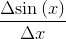Now here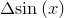has no unit as it is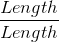and unit of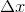is radians.
So the unit of the slope of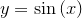is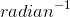. But here slope of the graph is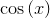which has no unit. So how could it be possible that a value with no unit is equal to a value with a unit?

16. Murray says:

@Rohan: Interesting point! I think this goes into the category of the slope of sin(x) at the point x "is numerically equal to" the cosine of x at the point x.

It's a bit like the flexibility of radians - they are really a measure of angle (an "amount of turn") but can be thought of as a distance around an arc, and hence their usefulness in calculus.

### Comment Preview

HTML: You can use simple tags like <b>, <a href="...">, etc.

To enter math, you can can either:

1. Use simple calculator-like input in the following format (surround your math in backticks, or qq on tablet or phone):
a^2 = sqrt(b^2 + c^2)
(See more on ASCIIMath syntax); or
2. Use simple LaTeX in the following format. Surround your math with $$ and $$.
$$\int g dx = \sqrt{\frac{a}{b}}$$
(This is standard simple LaTeX.)

NOTE: You can mix both types of math entry in your comment.